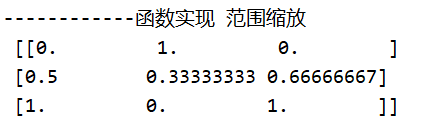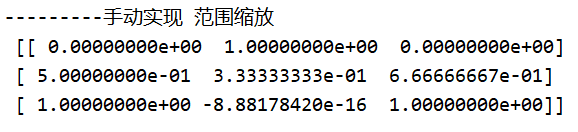• 如何缩放到初始绘图/缩放的特定范围
2021-03-06 22:30:45

您可以在figure()调用的最开始处特别设置所需的范围。。。让我们看一个例子：import numpy as np

from bokeh.sampledata.stocks import AAPL, FB, GOOG, IBM, MSFT

from bokeh.plotting import *

output_file("stocks.html", title="stocks.py example")

left_range = np.datetime64('2000-03-01')

right_range = np.datetime64('2006-03-01')

p1 = figure(x_axis_type = "datetime", x_range=[left_range, right_range])

p1.title = "Stock Closing Prices"

p1.grid.grid_line_alpha=0.3

p1.xaxis.axis_label = 'Date'

p1.yaxis.axis_label = 'Price'

show(p1)

这里，我刚刚定义了范围的左值和右值：

^{pr2}$如果你需要更多的帮助，请告诉我。在 更多相关内容 • 经过一番资料搜索，不光解决了这个问题还找到了对数缩放的方法，接下来就让我们一起看看吧~坐标轴最小和最大值为了在图表上显示特定区域，可以手动设置坐标轴的最小值和最大值。from openpyxl import Workbookfrom ... 在使用openpyxl时，坐标轴的调整就难住了小编。经过一番资料搜索，不光解决了这个问题还找到了对数缩放的方法，接下来就让我们一起看看吧~ 坐标轴最小和最大值 为了在图表上显示特定区域，可以手动设置坐标轴的最小值和最大值。from openpyxl import Workbook from openpyxl.chart import ( ScatterChart, Reference, Series, ) wb = Workbook() ws = wb.active ws.append(['X', '1/X']) for x in range(-10, 11): if x: ws.append([x, 1.0 / x]) chart1 = ScatterChart() chart1.title = "Full Axes" chart1.x_axis.title = 'x' chart1.y_axis.title = '1/x' chart1.legend = None chart2 = ScatterChart() chart2.title = "Clipped Axes" chart2.x_axis.title = 'x' chart2.y_axis.title = '1/x' chart2.legend = None chart2.x_axis.scaling.min = 0 chart2.y_axis.scaling.min = 0 chart2.x_axis.scaling.max = 11 chart2.y_axis.scaling.max = 1.5 x = Reference(ws, min_col=1, min_row=2, max_row=22) y = Reference(ws, min_col=2, min_row=2, max_row=22) s = Series(y, xvalues=x) chart1.append(s) chart2.append(s) ws.add_chart(chart1, "C1") ws.add_chart(chart2, "C15") wb.save("minmax.xlsx")在某些情况下，如上面代码所示，设置坐标轴范围实际上等同于显示数据的子范围。对于大型数据集，使用Excel或者Open/Libre Office来绘制散点图(可能还有其他)时，选择数据子集方式要比设置坐标轴范围的速度更快。 对数缩放 x轴和y轴都可以对数缩放。对数的基可以设置为任何有效的浮点。如果x轴按对数缩放，则将丢弃区域中的负值。from openpyxl import Workbook from openpyxl.chart import ( ScatterChart, Reference, Series, ) import math wb = Workbook() ws = wb.active ws.append(['X', 'Gaussian']) for i, x in enumerate(range(-10, 11)): ws.append([x, "=EXP(-(($A${row}/6)^2))".format(row = i + 2)]) chart1 = ScatterChart() chart1.title = "No Scaling" chart1.x_axis.title = 'x' chart1.y_axis.title = 'y' chart1.legend = None chart2 = ScatterChart() chart2.title = "X Log Scale" chart2.x_axis.title = 'x (log10)' chart2.y_axis.title = 'y' chart2.legend = None chart2.x_axis.scaling.logBase = 10 chart3 = ScatterChart() chart3.title = "Y Log Scale" chart3.x_axis.title = 'x' chart3.y_axis.title = 'y (log10)' chart3.legend = None chart3.y_axis.scaling.logBase = 10 chart4 = ScatterChart() chart4.title = "Both Log Scale" chart4.x_axis.title = 'x (log10)' chart4.y_axis.title = 'y (log10)' chart4.legend = None chart4.x_axis.scaling.logBase = 10 chart4.y_axis.scaling.logBase = 10 chart5 = ScatterChart() chart5.title = "Log Scale Base e" chart5.x_axis.title = 'x (ln)' chart5.y_axis.title = 'y (ln)' chart5.legend = None chart5.x_axis.scaling.logBase = math.e chart5.y_axis.scaling.logBase = math.e x = Reference(ws, min_col=1, min_row=2, max_row=22) y = Reference(ws, min_col=2, min_row=2, max_row=22) s = Series(y, xvalues=x) chart1.append(s) chart2.append(s) chart3.append(s) chart4.append(s) chart5.append(s) ws.add_chart(chart1, "C1") ws.add_chart(chart2, "I1") ws.add_chart(chart3, "C15") ws.add_chart(chart4, "I15") ws.add_chart(chart5, "F30") wb.save("log.xlsx") 这将生成五个类似的图表：五张图使用了相同的数据。其中，第一个图未缩放，第二和三张图分别缩放了X和Y轴，第四张图XY轴均进行了缩放，对数基数设置为10；最后的图表XY轴均进行了缩放，但对数的底设置为e。 轴线方向 坐标轴可以正常显示，也可以反向显示。 轴方向由orientation属性控制，minMax表示正向，maxMin表示反向。from openpyxl import Workbook from openpyxl.chart import ( ScatterChart, Reference, Series, ) wb = Workbook() ws = wb.active ws["A1"] = "Archimedean Spiral" ws.append(["T", "X", "Y"]) for i, t in enumerate(range(100)): ws.append([t / 16.0, "=$A${row}*COS($A${row})".format(row = i + 3), "=$A${row}*SIN($A\${row})".format(row = i + 3)])

chart1 = ScatterChart()

chart1.title = "Default Orientation"

chart1.x_axis.title = 'x'

chart1.y_axis.title = 'y'

chart1.legend = None

chart2 = ScatterChart()

chart2.title = "Flip X"

chart2.x_axis.title = 'x'

chart2.y_axis.title = 'y'

chart2.legend = None

chart2.x_axis.scaling.orientation = "maxMin"

chart2.y_axis.scaling.orientation = "minMax"

chart3 = ScatterChart()

chart3.title = "Flip Y"

chart3.x_axis.title = 'x'

chart3.y_axis.title = 'y'

chart3.legend = None

chart3.x_axis.scaling.orientation = "minMax"

chart3.y_axis.scaling.orientation = "maxMin"

chart4 = ScatterChart()

chart4.title = "Flip Both"

chart4.x_axis.title = 'x'

chart4.y_axis.title = 'y'

chart4.legend = None

chart4.x_axis.scaling.orientation = "maxMin"

chart4.y_axis.scaling.orientation = "maxMin"

x = Reference(ws, min_col=2, min_row=2, max_row=102)

y = Reference(ws, min_col=3, min_row=2, max_row=102)

s = Series(y, xvalues=x)

chart1.append(s)

chart2.append(s)

chart3.append(s)

chart4.append(s)

wb.save("orientation.xlsx")

这将生成四个图表，其中每个可能的方向组合的轴如下所示：小伙伴们可以根据自己的需求，生成不同的图表~如需了解更多python实用知识，点击进入PyThon学习网教学中心。

展开全文• python sklearn 数据预处理 范围缩放 """ 数据预处理 最大最小值缩放 """ import numpy as np import sklearn.preprocessing as sp # 准备数据 raw_samples = np.array([ [17, 90, 4000], [20, 80, 5000], [23, ...

## python sklearn 数据预处理 范围缩放

"""
数据预处理 最大最小值缩放
"""
import numpy as np
import sklearn.preprocessing as sp

# 准备数据
raw_samples = np.array([
[17, 90, 4000],
[20, 80, 5000],
[23, 75, 5500]
])

# 使用函数 范围缩放
mms = sp.MinMaxScaler(feature_range=(0, 1))
result = mms.fit_transform(raw_samples)
print("------------函数实现 范围缩放\n", result)

# 手动实现 范围缩放
new_samples = []
for row in raw_samples.T:   # 转置 对列处理
min_val = np.min(row)
max_val = np.max(row)

# 整理解方程需要的 A， B  线性回归解方程
A = np.array([[min_val, 1], [max_val, 1]])
B = np.array([0, 1])

x = np.linalg.solve(A, B)    # 求解结果 x= k x=b
new_row = row * x + x  # y = kx + b
new_samples.append(new_row)

print("---------手动实现 范围缩放\n", np.array(new_samples).T)  # 再次转置 显示结果展开全文• 机器学习中的数据转换-Python Scikit-Learn实现方法...一、数据缩放你的预处理数据集中可能包含各种各样、不同比例的特征字段，比如美元、千克、价格，有的特征列数值范围在100以内，有的却达到几百万，差异很大。...

机器学习中的数据转换-Python Scikit-Learn实现方法

我们在建模之前，需要做数据准备。数据准备的过程主要包括3个步骤：数据选择

数据预处理

数据转换

本文会告诉你两种方法，即如何使用Python的Scikit-Learn库进行简单的数据转换。

一、数据缩放

你的预处理数据集中可能包含各种各样、不同比例的特征字段，比如美元、千克、价格，有的特征列数值范围在100以内，有的却达到几百万，差异很大。

很多机器学习模型不希望有这样大的差异，如果数据集各个属性之间的数值差异越小，则模型表现的会越好。这里不一概而论，具体原因小伙伴们自行探索下。

方法1：数据归一化

数据归一化是指将原始数据缩放到0和1之间。

对于依赖于值的大小的模型，这种对输入属性的缩放很管用。比如在K近邻模型的距离测量和回归系数的准备。

接下来用大名鼎鼎的鸢尾花数据集演示数据归一化的例子。

# 对鸢尾花数据集进行数据归一化处理.

from sklearn import preprocessing

# 加载数据

print(iris.data.shape)

# 分离原始数据集，分为自变量和因变量

X = iris.data

y = iris.target

# 归一化处理

normalized_X = preprocessing.normalize(X)

更多关于归一化处理，详见API

方法2：数据标准化

数据标准化是指：数据集中，每个特征列的数值减去其平均值，再除以其标准差，得到均值为0，标准差为1的服从标准正态分布的数据。

诸如高斯过程等依赖于特征分布的模型，对特征进行标准化是非常有用的。

依然用鸢尾花的例子。

# 对鸢尾花数据集进行数据标准化处理.

from sklearn import preprocessing

# 加载数据

print(iris.data.shape)

# 分离原始数据集，分为自变量和因变量

X = iris.data

y = iris.target

# 标准化处理

standardized_X = preprocessing.scale(X)

更多关于归一化处理，详见API

二、说明

很难知道数据缩放是否会有利于模型的效果，大概率上是有用的，但不排除出现产生负效果。

中肯的建议是，先建立一个数据缩放后的副本，使用你的算法模型测试对比缩放前后的效果。

这可以让你快速知道，你的模型中缩放带来的好处或弊端。你也可以试用不同的缩放方法，进行对比。

三、总结

数据缩放是一种非常重要的数据转换方法。本文介绍了归一化和标准化两种方法，均用Python Scikit-Learn库提供的方法实现。简单好用，快试试吧！

展开全文• ## python数值大小缩放

千次阅读 2020-05-16 11:54:16
最近需要画一个networkx图，其中边的权重来决定线的粗细。原本数据相差特别大，这就导致有的线很粗有的超级细。 于是我们想把一组数据，映射到另一组，使得它们差距小一点。 这个里面其实有很多方法，比如归一化，...
• 多维缩放可以为数据集找到一种二维表达形式。算法根据每对数据项之间的差距情况，尝试绘制出一幅图来，图中的各数据项之间的距离远近，对应于它们彼此间的差异程度。以分类聚集算法中的数据为例第一步：计算所有数据...
• ## python QT 图片缩放，移动

千次阅读 多人点赞 2020-05-23 14:35:23
利用python的QT库搭建图片显示界面，实现图片的拖拽、以鼠标中心缩放等功能。数据可视化
• 特征缩放(feature scaling): 改变特征的取值范围缩放到统一的区间，例如[0,1][0, 1][0,1]. 为什么要进行特征缩放？ 数据集包含众多特征，每个特征的尺度(scale)不同，有的特征的单位是小时，有的特征的单位是公里...机器学习 人工智能 大数据
• 我只想绘制部分数组，固定x部分，但让y部分自动缩放。我尝试如下所示，但是它不起作用。有什么建议么？import numpy as npimport matplotlib.pyplot as pltdata=[np.arange(0,101,1),300-0.1*np.arange(0,101,1)]plt...
• python 机器学习之数据预处理与缩放 序 之前我们在接触监督学习时了解到，有一些算法（譬如神经网络和SVM）对于数据的缩放非常敏感。因此，通常的做法是对数据集进行调节，使得数据表示更适合于这些算法。通常来说，...数据挖掘 机器学习 svm 神经网络
• 文章目录前言基于 QChart 缩放的几种方式使用QChartView来缩放使用QChart来平移和缩放使用QValueAxis或者QDateTimeAxis来平移和缩放Qt曲线图表模块QChart库缩放、平移实例 前言 最近使用Qt for python 做了一个图表...
• 需求 参考官网：Scales — Matplotlib 3.4.3 documentation 常规画图时，有时候对X轴或者Y轴，希望某个区域可以放大，某个...X轴范围0-100，希望一半区域是0-10，另外一半是10-100 forward和inverse是比例转换及其..
• 有一个watermark example与...我这样做是因为extent参数会影响ax的最终范围.由于我们希望最终范围由plt.plot(…)控制,让我们把它放在最后.myaximage = ax.imshow(im, aspect='auto', extent=(1,15,0.3,0.7), al...
• 但可以借助python的opencv 要保证路径是全英文的，这很重要，文件可以不用中文，可以做一些自动名字重命名再改回原来名字的操作。 import os import cv2 import sys #定义图像缩放函数 def...
• 封面图片：《中学生可以这样学Python》，董付国，应根球著，清华大学出版社==============问题描述：创建窗体，显示一张图片，当改变窗体大小时，自动缩放并显示图片。参考代码：运...编程语言 大数据 github html
• import io from PIL import Image, ImageTk import tkinter as tk def resize(w, h, w_box, h_box, pil_image): ''' resize a pil_image object so it will fit into... 对一个pil_image对象进行缩放，让它在.tkinter 界面设计 图像识别 开发语言
• pil_image.size # resize the image so it retains its aspect ration # but fits into the specified display box #缩放图像让它保持比例，同时限制在一个矩形框范围内 pil_image_resized = resize(w, h, w_box, h_...
• 范围缩放 将样本矩阵中的每一列的最小值和最大值设定为相同的区间, 统一各列特征值的范围. 一般情况下,会把特征区间缩放至[0,1]. [17, 20, 23] 如何使这组数据的最小值等于0: [0, 3, 6] 如何使这组数据的最大值等于1...机器学习 人工智能
• 公司一个项目，在启动web页面时，默认应该是打开...　因为开发在手头工作量太大了，在浏览器适配方面就没有做好（开发是大哥，项目经理上了都不顶用），于是我们的项目只有在浏览器缩放到90%情况下，页面内容才能优...
• image对象进行缩放，让它在一个矩形框内，还能保持比例 ''' f1 = 1.0*w_box/w # 1.0 forces float division in Python2 f2 = 1.0*h_box/h factor = min([f1, f2]) #print(f1, f2, factor) # test # use best down-...
• Python OpenCV 图片的读取、缩放，裁剪与保存 import numpy as np import cv2 as cv ** 读取图片 ** original = cv.imread('p4.jpg') print(original.shape) cv.imshow('1',original) blue = np.zeros_like(original...
• 1、什么是范围缩放 统一样本矩阵中的不同特征的最大值和最小值的范围。（一般都是0-1） 例如设样本集为X [0, 1, 2, 3,4, 5 ] 范围缩放到【0-1】后 X1 [0, 0.2, 0.4, 0.6, 0.8, 1] kmin+ b = min’ =0 原本...机器学习 人工智能
• Q4 哪些数据不能做特征缩放？二、特征标准化/方差放缩三、特征归一化1. min-max缩放2. L2归一化3. 十进制归一化四、实例——不同缩放方法的对比 一、Q&A Q1 什么是标准化、归一化、规范化、正则化？...
• 在机器学习过程中，对数据的处理过程中，常常需要对数据进行归一化处理，下面介绍（0， 1）标准化的方式，简单的说，其功能就是将预处理的数据的数值范围按一定关系“压缩”到（0，1）的范围类。 通常（0， 1）标注...
•点云 刚体变换
• 在本例中，只有-0.5到1.5之间的范围显示在条形图中，而颜色图覆盖-2到2（因此这可能是您的数据范围，您在缩放之前记录的数据范围）。 因此，不要缩放颜色映射，而是缩放数据并使颜色条与之匹配。 参考回复 使用图形......